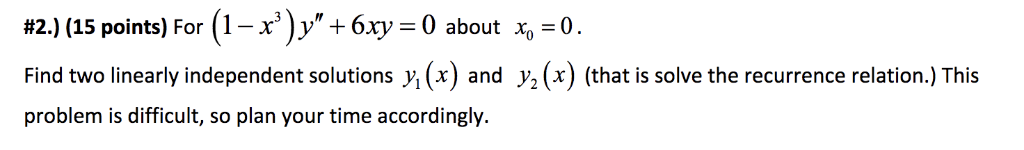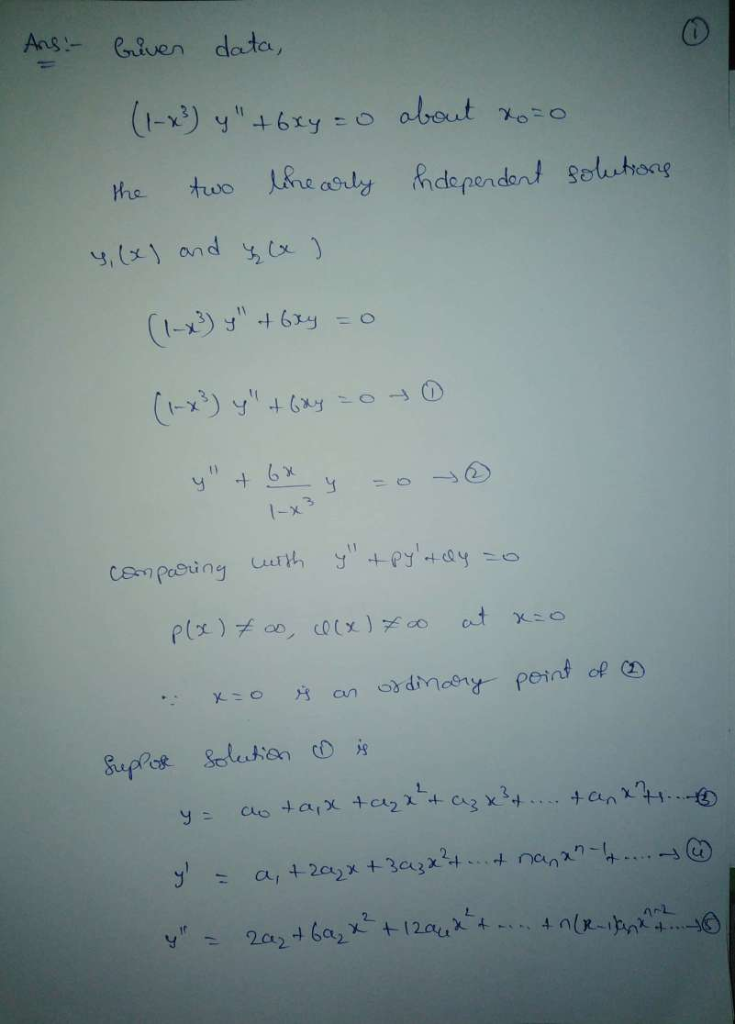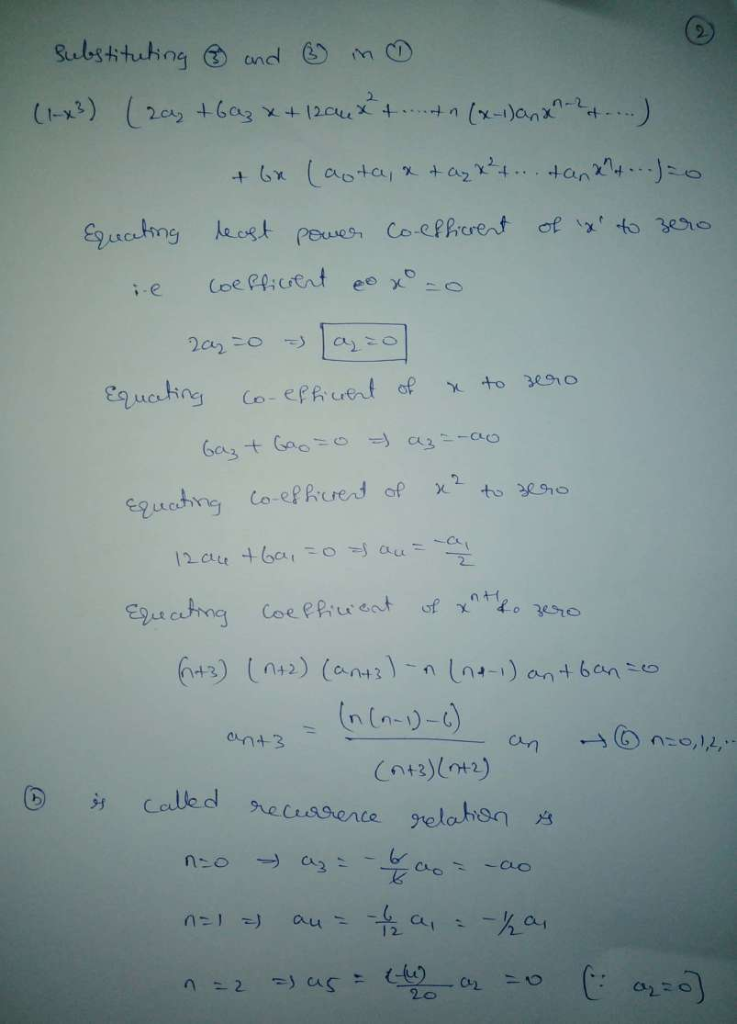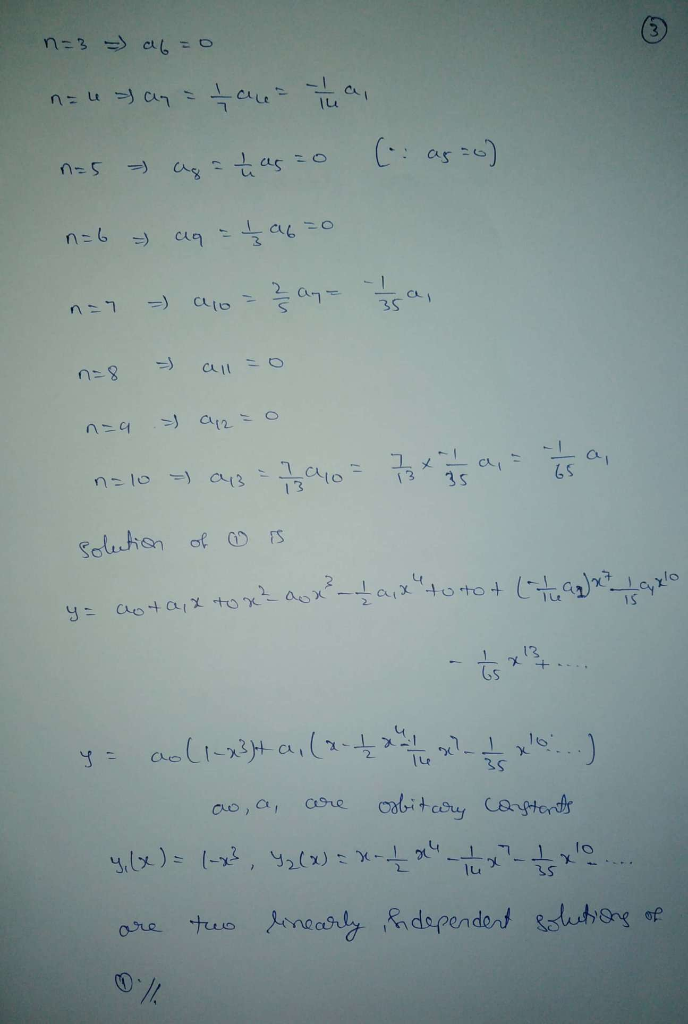# ' )y" + 6xy = 0 about x。:0. #2.) (15 points) For ( 1-X Find two linearly independent solutions y,(x) and V2(x)...' )y" + 6xy = 0 about x。:0. #2.) (15 points) For ( 1-X Find two linearly independent solutions y,(x) and V2(x) (that is solve the recurrence relation.) This problem is difficult, so plan your time accordingly##### Add Answer of: ' )y" + 6xy = 0 about x。:0. #2.) (15 points) For ( 1-X Find two linearly independent solutions y,(x) and V2(x)...
More Homework Help Questions Additional questions in this topic.